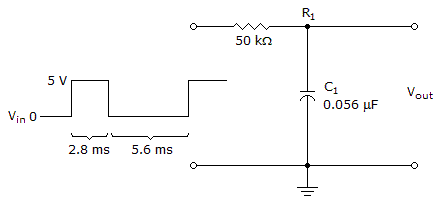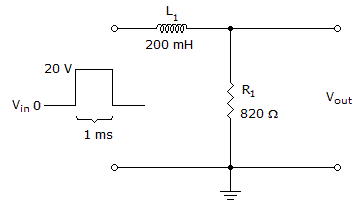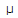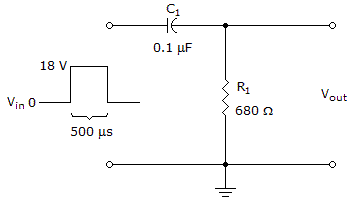# Electronics - Time Response of Reactive Circuits

### Exercise :: Time Response of Reactive Circuits - Filling the Blanks

1.The output voltage in the given circuit is _____ at the end of the second pulse.

 A. 1.06 V B. 7.56 V C. 3.32 V D. 12 V

Answer: Option C

Explanation:

No answer description available for this question. Let us discuss.

2.It takes ______ for the output voltage in the given circuit to reach its maximum value.

 A. 0 s B. 243.9s C. 1 ms D. 1.22 ms

Answer: Option D

Explanation:

No answer description available for this question. Let us discuss.

3.

For a capacitor to completely charge in an RC integrator, the pulse width must be _____ 5 time constants.

 A. less than B. greater than C. equal to D. greater than or equal to

Answer: Option D

Explanation:

No answer description available for this question. Let us discuss.

4.

The _____ is the time it takes for a capacitor to fully charge or discharge.

 A. transient time B. pulse response C. time constant D. steady state

Answer: Option A

Explanation:

No answer description available for this question. Let us discuss.

5.After the rising edge of the input pulse occurs, it takes ______ for the voltage across the resistor to decrease to zero in the given circuit.

 A. 0 s B. 68s C. 340s D. 500s

Answer: Option C

Explanation:

No answer description available for this question. Let us discuss.

#### Current Affairs 2021

Interview Questions and Answers